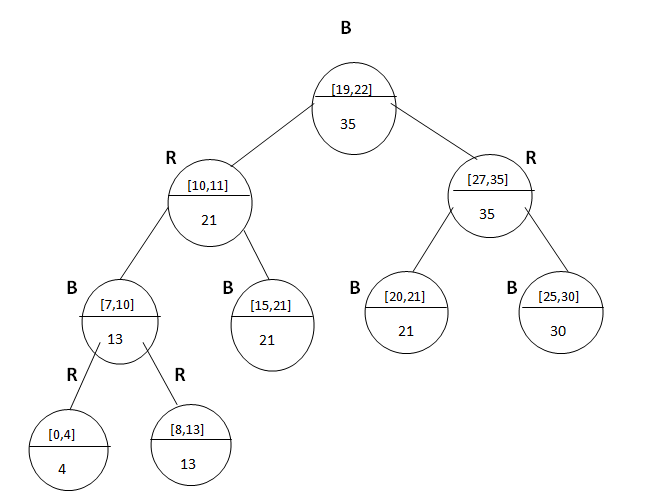# Interval Tree in Data Structure

In this article, we are going to discuss about the interval Tree, algorithm for searching in Interval tree and the augment of interval tree in data structure.
Submitted by Prerana Jain, on July 19, 2018

## Interval Tree

Interval tree is a Red-black Tree in which each node has an interval represented by an ordered pair [t1, t2] such that t1 < t2.

Here, t1 is called lower end point and t2 is called higher end point. If both the endpoints are mentioned in the nodes of the tree than it is called closed Interval tree. If we omit both the ends points then it is called an open interval tree and if we omit one end of the endpoints from the set then it is called half-open interval. In this section, we will discuss an interval tree with a closed interval.

The interval tree is a useful data structure for the database of real-time applications, the interval tree is a useful data structure for representing the events.

If the interval [t1,t2] is for an object i then low [i] = t1 and high [i] = t2. There could be another interval tree t2 which is overlapping with t1 or t2. The interval tree for the following set of the interval,

If there are two intervals i and i' the following conditions can be possible:

1. If i and i' overlap then low[i] <= high [i'] and low [i'] <= high[i].
2. If there are no overlapping intervals then high[i] < low[i'].
3. If the interval do not overlap high [i'] < low[i].

### Augment of Interval Tree

let us now augment the interval tree data structure.

Step 1) Choose the data structure which has to be augmented.

We will choose a red-black tree with intervals. The inorder traversal of interval tree with low-end point gives the key value of each node.

Step 2) find out the additional information that is needed to support the algorithm.

Each node K contain a value max[k] which is the maximum value of any interval endpoints. This maximum value can be obtained using the following formula.

` Max [k] = max(high[int[k]]), max[left[k]], max[right[k]])`

The interval tree can be shown with augmented data structure as:Consider a root node [19, 22] for which we want to find max value then:

```    =   max(high (node [19,22]), max(left[19,22])), max(right[27,35])))
=   max (22, 21, 35)
=   35
```

Hence, in the max field of root node we put the value 35.

Step 3) Verification of additional information

With this additional field of max, the insertion and deletion operation do not lose their performance efficiency. The insertion and deletion can be performed in O(log n).

Step 4) Develop a new operation for additional information.

Using the additional information max field we can search the desired node in interval tree using the following algorithm.

## Algorithm for searching in Interval Tree

INTERVAL SEARCH (T, i) - returns a pointer to an element X in the interval tree t such that int [x] overlap i1 on the sentinel nil [T] if no such element is in the set.

```    INTERVAL SEARCH (T, i)
1.	 x <- root [T]
2.	 While x is not equals nil [T] and i does not overlap int [x].
3.	 Do if left [x] is not equal to nil [T] and max [left (x)] >= low [i].
4.	Then x <- left [x].
5.	Else x <- right [x]
6.	Return x.
```

### Analysis

If the height of the red-black tree is n than each operation takes O(1) time and the whole procedure searching will be executed in O(log n) time. Hence, time complexity of this algorithm is O(log n).

Top MCQs

Languages: » C » C++ » C++ STL » Java » Data Structure » C#.Net » Android » Kotlin » SQL
Web Technologies: » PHP » Python » JavaScript » CSS » Ajax » Node.js » Web programming/HTML
Solved programs: » C » C++ » DS » Java » C#
Aptitude que. & ans.: » C » C++ » Java » DBMS
Interview que. & ans.: » C » Embedded C » Java » SEO » HR
CS Subjects: » CS Basics » O.S. » Networks » DBMS » Embedded Systems » Cloud Computing
» Machine learning » CS Organizations » Linux » DOS
More: » Articles » Puzzles » News/Updates